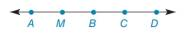Chapter 1.3, Problem 14E### Elementary Geometry for College St...

6th Edition
Daniel C. Alexander + 1 other
ISBN: 9781285195698

#### Solutions

Chapter
Section### Elementary Geometry for College St...

6th Edition
Daniel C. Alexander + 1 other
ISBN: 9781285195698
Textbook Problem
1 views

# In Exercises 9 to 28, use the drawings as needed to answer the following questions.Classify as true or false: a) A B + B C = A D d) A B + B C + C D = A D b) A D − C D = A B e) A B = B C c) A D − C D = A CExercises 14 − 17

To determine

a)

To check:

Whether the given statement is true or false.

Explanation

Given:

The given figure is,

And, the given statement is,

Approach:

Consider the given figure,

Figure (1)

From the figure (1), AB=2units and BC=1unit.

To determine

b)

To check:

Whether the given statement is true or false.

To determine

c)

To check:

Whether the given statement is true or false.

To determine

d)

To check:

Whether the given statement is true or false.

To determine

e)

To check:

Whether the given statement is true or false.

### Still sussing out bartleby?

Check out a sample textbook solution.

See a sample solution

#### The Solution to Your Study Problems

Bartleby provides explanations to thousands of textbook problems written by our experts, many with advanced degrees!

Get Started

#### Find the limit (if possible): limx25x+2.

Calculus: An Applied Approach (MindTap Course List)

#### State the domain and range of each of the function in Problems 25 – 28. 28. .

Mathematical Applications for the Management, Life, and Social Sciences

#### True or False: , for –π ≤ t ≤ π, is smooth.

Study Guide for Stewart's Multivariable Calculus, 8th

#### If f(x) = 2e3x, f(x) = a) 2e3x b) 2xe3x c) 6e3x d) 6xe3x

Study Guide for Stewart's Single Variable Calculus: Early Transcendentals, 8th